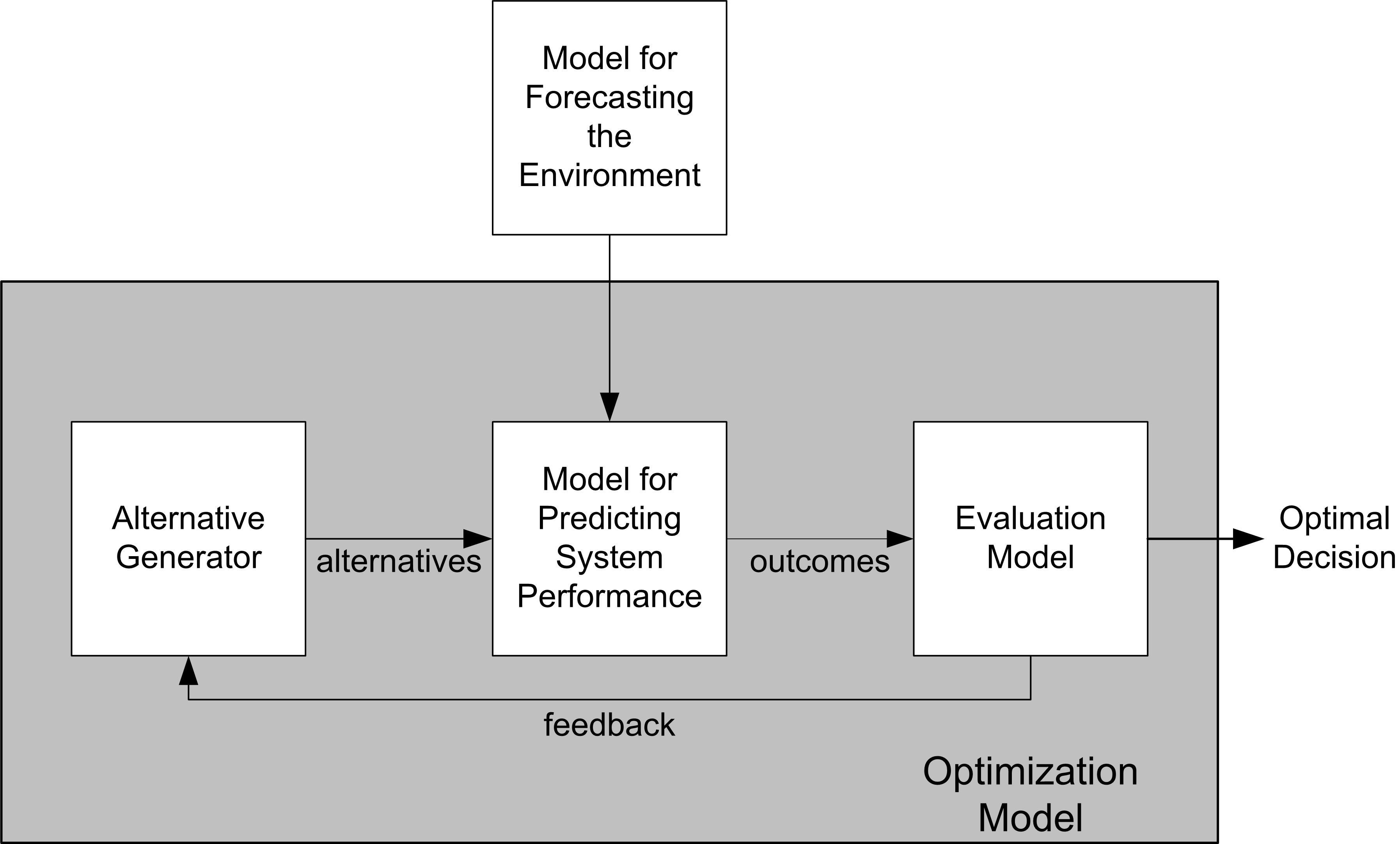## 1.4 Simulation: Descriptive or Prescriptive Modeling?

A descriptive model describes how a system behaves. Simulation is at its heart a descriptive modeling technique. Simulation is used to depict the behaviors or characteristics of existing or proposed systems. However, a key use of simulation is to convey the required behaviors or properties of a proposed system. In this situation, simulation is used to prescribe a solution. A prescriptive model tells us what to do. In other words, simulation can also be used for prescriptive modeling. Figure 1.3 illustrates the concept of using simulation to recommend a solution.Figure 1.3: Using simulation for prescriptive analysis

In the figure, a simulation model is used for predicting the behavior of the system. Input models are used to characterize the system and its environment. An evaluative model is used to evaluate the output of the simulation model to understand how the output compares to desired goals. The alternative generator is used to generate different scenarios to be feed into the simulation model for evaluation. Through a feedback mechanism the inputs can be changed based on the evaluation of the outputs and eventually a recommended solution can be achieved.

For example, in the modeling a drive up pharmacy, suppose that the probability of a customer waiting longer than 3 minutes in line had to be less than 10%. To form design alternatives, the inputs (e.g. number of pharmacists, possibly the service process) can be varied. Each alternative can then be evaluated to see if the waiting time criteria is met. In this simple situation, you might act as your own alternative generator and the evaluative model is as simple as meeting a criteria; however, in more complex models, there will often be hundreds of inputs to vary and multiple competing objectives. In such situations, simulation optimization and heuristic search methods are often used. This is an active and important area of research within simulation.